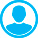ON THE EFFECTS OF THE INERTIA ELLIPSOID TRIAXIALITY IN THE THEORY OF NUTATIONAbstract (English):
Modern nutation theories of the Earth and planets use the assumption, that the planetҳ inertia ellipsoid is symmetrical about the axes of rotation. Below we estimate the effects of the inertia ellipsoid triaxiality accurate to B-A/C-A4, where A, B, C are equatorial and polar principal moments of inertia, respectively.

Keywords:
theory of nutation, inertia ellipsoid triaxiality, Earth tides.